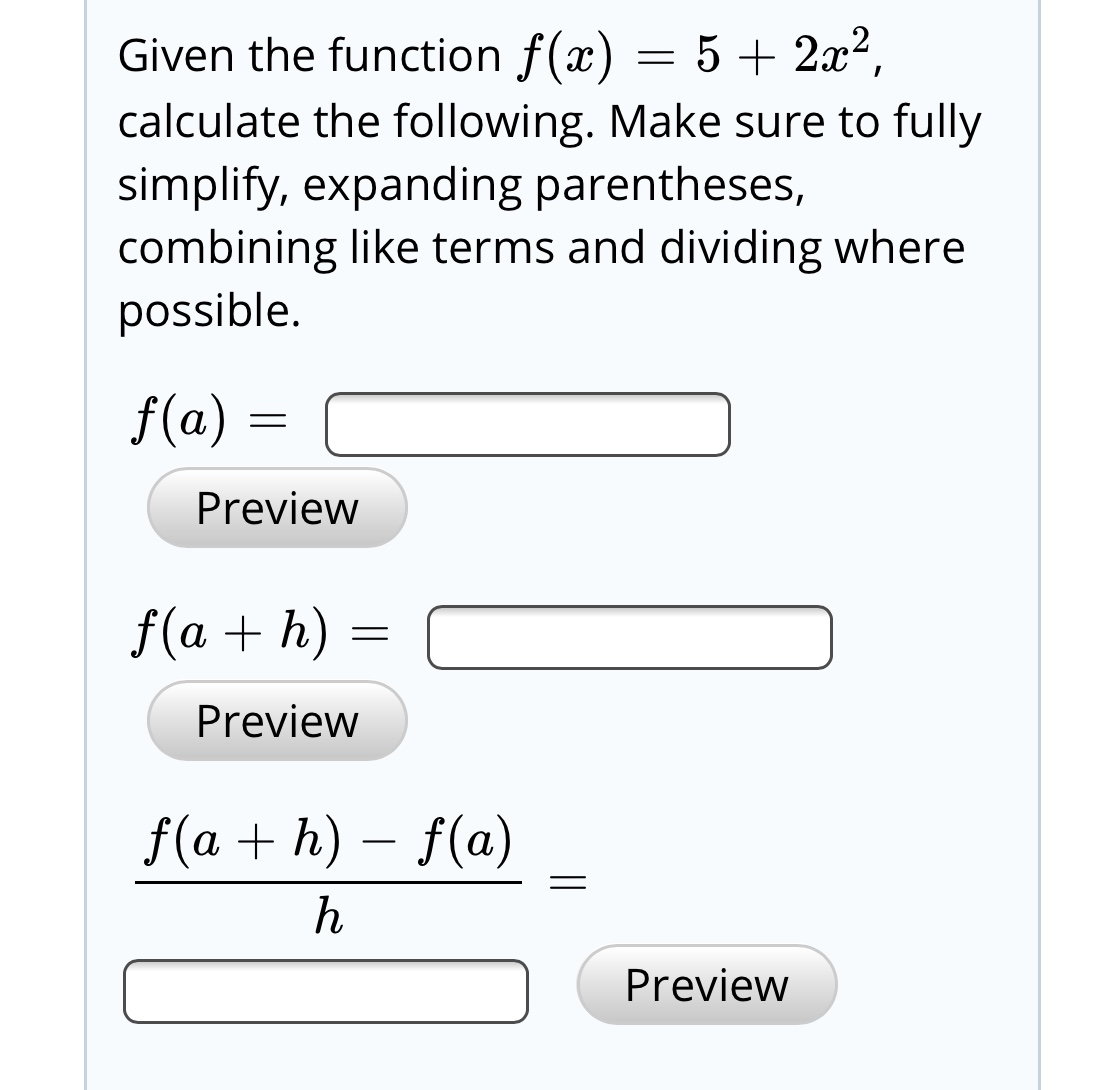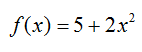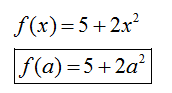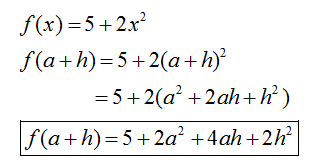# Given the function f(x) = 5 + 2x²,calculate the following. Make sure to fullysimplify, expanding parentheses,combining like terms and dividing wherepossible.f(a)Previewf(a + h) =Previewf(a + h) – f(a)Preview

Question
1 viewshelp_outlineImage TranscriptioncloseGiven the function f(x) = 5 + 2x², calculate the following. Make sure to fully simplify, expanding parentheses, combining like terms and dividing where possible. f(a) Preview f(a + h) = Preview f(a + h) – f(a) Preview fullscreen
check_circle

Step 1

Given the function:Step 2

First, we find f (a)Step 3

Now, we find f (a + h)...

### Want to see the full answer?

See Solution

#### Want to see this answer and more?

Solutions are written by subject experts who are available 24/7. Questions are typically answered within 1 hour.*

See Solution
*Response times may vary by subject and question.
Tagged in